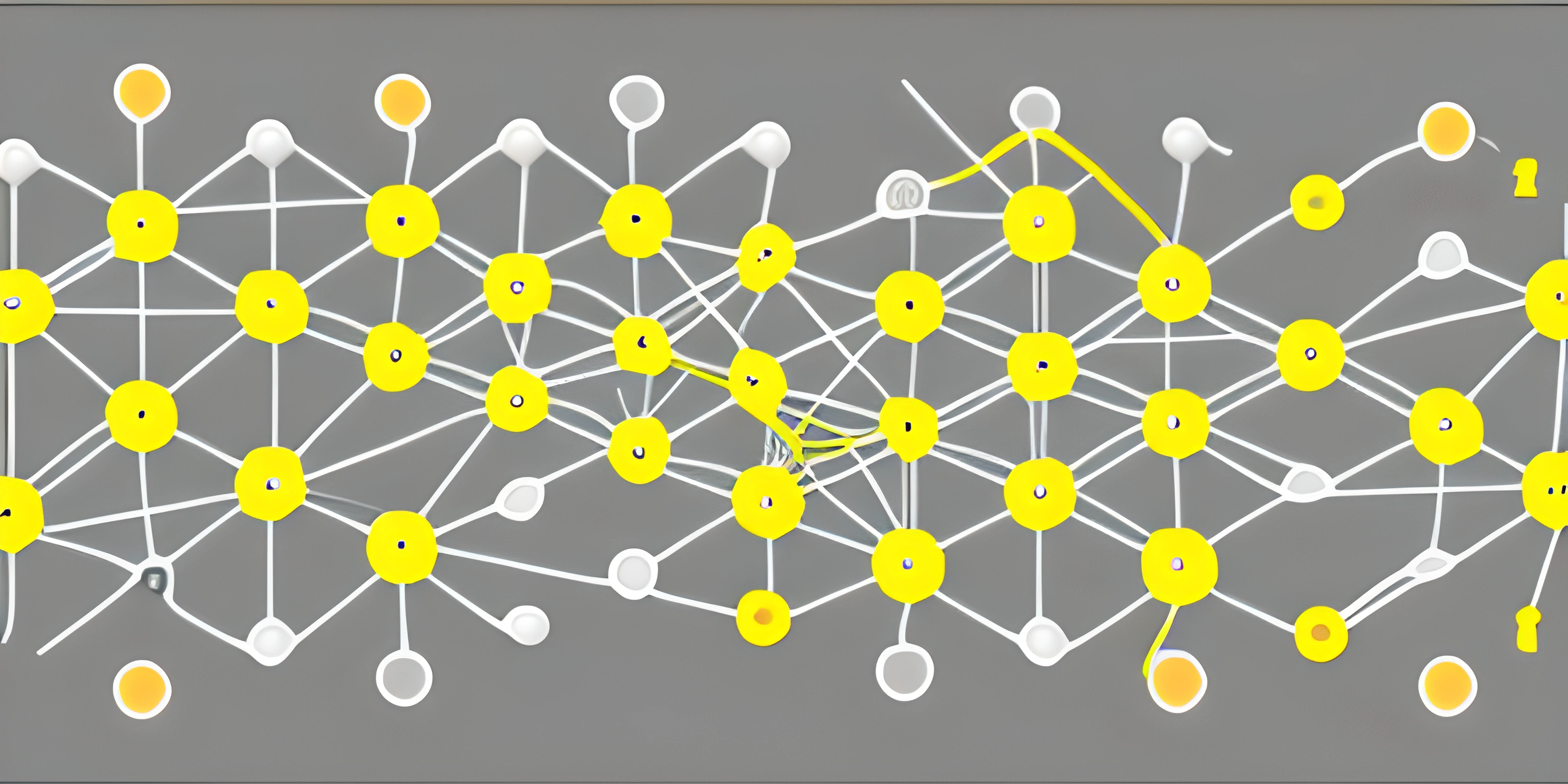# Dijkstra's AlgorithmNote: this page has been created with the use of AI. Please take caution, and note that the content of this page does not necessarily reflect the opinion of Cratecode.

Dijkstra's algorithm is like the GPS of programming – it helps us navigate through a network of nodes, finding the shortest path along the way. Named after its creator, Edsger Dijkstra, this powerful algorithm is used to solve a variety of problems that involve finding the quickest route or the least cost path in a graph.

## Understanding Graphs

Imagine you're playing a game of Pac-Man, and you need to collect all the pellets as quickly as possible. In this scenario, the game board can be represented as a graph, with nodes (pellets) connected by edges (paths). The goal is to find the shortest path that touches each node exactly once. That's where Dijkstra's algorithm comes in handy.

A graph is a collection of nodes (also called vertices) and edges that connect them. Nodes can represent anything from cities in a map to web pages in the internet. Edges, on the other hand, symbolize the connections between these nodes, often with an associated weight or cost (like distance or time).

## Dijkstra's Algorithm

Dijkstra's algorithm is a greedy algorithm that finds the shortest path from a starting node to all other nodes in a graph. The algorithm maintains a set of unvisited nodes and a current distance value for each node. Initially, the distance to the starting node is set to 0, while the distances to all other nodes are set to infinity. Dijkstra's algorithm follows these steps:

1. Select the node with the smallest distance (initially the starting node) and mark it as visited.
2. Update the distances of neighboring nodes by considering the weight of the edge connecting the current node to its neighbors.
3. Repeat steps 1 and 2 for all unvisited nodes until all nodes have been visited or the target node has been visited.

Here's a high-level pseudocode representation of Dijkstra's algorithm:

``````function dijkstra(graph, start):
distances = {}
visited = set()

# Initialize distances
for node in graph.nodes:
distances[node] = float("inf")
distances[start] = 0

# Process the nodes
while len(visited) < len(graph.nodes):
# Find the node with the smallest distance that has not been visited
min_node = None
for node in graph.nodes:
if node not in visited and (min_node is None or distances[node] < distances[min_node]):
min_node = node

# Update the distances of neighboring nodes
for neighbor, weight in graph.edges[min_node]:
new_distance = distances[min_node] + weight
if new_distance < distances[neighbor]:
distances[neighbor] = new_distance